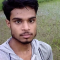Class Central is learner-supported. When you buy through links on our site, we may earn an affiliate commission.

# Machine Learning with Python

via freeCodeCamp

### Overview

Machine learning has many practical applications that you can use in your projects or on the job.

In the Machine Learning with Python Certification, you'll use the TensorFlow framework to build several neural networks and explore more advanced techniques like natural language processing and reinforcement learning.

You'll also dive into neural networks, and learn the principles behind how deep, recurrent, and convolutional neural networks work.

Major Topics:
• TensorFlow
• How Neural Networks Work
• Machine Learning with Python Projects

### Syllabus

• Introduction: Machine Learning Fundamentals
• Introduction to TensorFlow
• Core Learning Algorithms
• Core Learning Algorithms: Working with Data
• Core Learning Algorithms: Training and Testing Data
• Core Learning Algorithms: The Training Process
• Core Learning Algorithms: Classification
• Core Learning Algorithms: Building the Model
• Core Learning Algorithms: Clustering
• Core Learning Algorithms: Hidden Markov Models
• Core Learning Algorithms: Using Probabilities to make Predictions
• Neural Networks with TensorFlow
• Neural Networks: Activation Functions
• Neural Networks: Optimizers
• Neural Networks: Creating a Model
• Convolutional Neural Networks
• Convolutional Neural Networks: The Convolutional Layer
• Creating a Convolutional Neural Network
• Convolutional Neural Networks: Evaluating the Model
• Convolutional Neural Networks: Picking a Pretrained Model
• Natural Language Processing With RNNs
• Natural Language Processing With RNNs: Part 2
• Natural Language Processing With RNNs: Recurring Neural Networks
• Natural Language Processing With RNNs: Sentiment Analysis
• Natural Language Processing With RNNs: Making Predictions
• Natural Language Processing With RNNs: Create a Play Generator
• Natural Language Processing With RNNs: Building the Model
• Natural Language Processing With RNNs: Training the Model
• Reinforcement Learning With Q-Learning
• Reinforcement Learning With Q-Learning: Part 2
• Reinforcement Learning With Q-Learning: Example
• Conclusion
• How Deep Neural Networks Work
• Recurrent Neural Networks RNN and Long Short Term Memory LSTM
• Deep Learning Demystified
• How Convolutional Neural Networks work
• Rock Paper Scissors
• Cat and Dog Image Classifier
• Book Recommendation Engine using KNN
• Linear Regression Health Costs Calculator
• Neural Network SMS Text Classifier

### Taught by

freeCodeCamp Team

## Reviews

4.5 rating, based on 2 Class Central reviews

Start your review of Machine Learning with Python

• Hafedh Hedhly
This is best introductory programming course in python. Mentors are planting every concept in our head in very fluent ways. You must take take this course as a begineer python programmer like me.
•Rohit Pandey
This program is very excellent for any beginner and also a professional working and also Envolve advance course

### Never Stop Learning.

Get personalized course recommendations, track subjects and courses with reminders, and more.